# A long solenoid (cross-sectional area = 1.10*10^-6 m^2, number of turns per unit length = 2544...

A long solenoid (cross-sectional area = 1.10*10^-6 m^2, number of turns per unit length = 2544 turns/m) is bent into a circular shape so it looks like a donut. This wire-wound donut is called a toroid. Assume that the diameter of the solenoid is small compared to the radius of the toroid, which is 0.052 m. Find the emf induced in the toroid when the current decreases to 1.3 A from 2.7 A in a time of 0.14 s.

The answer is 2.92*10^-5. Just need to know the steps to solve. Thanks!

e = -L*dI/dt

we know

L = uo*n^2*A*length

length = circumference of circle = 2pi*R

n = 2544 turns/m

A = 1.10 x 10^-6

R = 0.052 m

dI = (1.3 - 2.7) = -1.4

dt = 0.14

uo = 4pi x 10^-7

e = 2.92 x 10^-7 V

#### Earn Coin

Coins can be redeemed for fabulous gifts.

Similar Homework Help Questions
• ### A long solenoid (cross-sectional area = 0.79 x 10-6 m2, number of turns per unit length...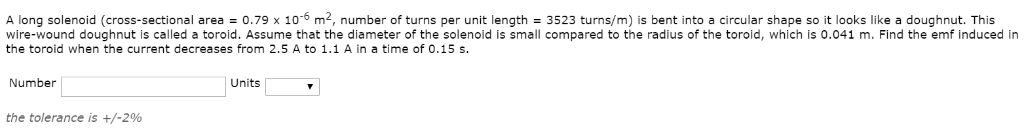A long solenoid (cross-sectional area = 0.79 x 10-6 m2, number of turns per unit length = 3523 turns/m) is bent into a circular shape so it looks like a doughnut. This wire-wound doughnut is called a toroid. Assume that the diameter of the solenoid is small compared to the radius of the toroid, which is 0.041 m. Find the emf induced in the toroid when the current decreases from 2.5 A to 1.1 A in a time of 0.15...

• ### A very long, straight solenoid with a cross-sectional area of 2.03 cm² is wound with 94.3...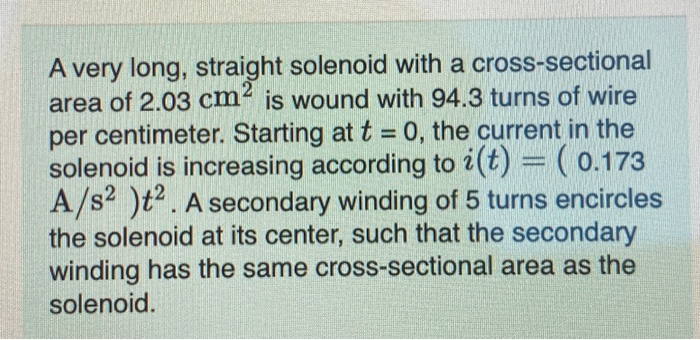A very long, straight solenoid with a cross-sectional area of 2.03 cm² is wound with 94.3 turns of wire per centimeter. Starting at t = 0, the current in the solenoid is increasing according to 2(t) = ( 0.173 A/s2 )t2. A secondary winding of 5 turns encircles the solenoid at its center, such that the secondary winding has the same cross-sectional area as the solenoid. What is the magnitude of the emf induced in the secondary winding at the...

• ### A very long, straight solenoid with a cross-sectional area of 1.92 cm2cm2 is wound with 86.9...

A very long, straight solenoid with a cross-sectional area of 1.92 cm2cm2 is wound with 86.9 turns of wire per centimeter. Starting at tt = 0, the current in the solenoid is increasing according to i(t)=i(t)= (( 0.164 A/s2A/s2 )t2)t2. A secondary winding of 5 turns encircles the solenoid at its center, such that the secondary winding has the same cross-sectional area as the solenoid. Part A What is the magnitude of the emf induced in the secondary winding at...

• ### A very long, straight solenoid with a cross-sectional area of 1.90 cm2 is wound with 89.7...

A very long, straight solenoid with a cross-sectional area of 1.90 cm2 is wound with 89.7 turns of wire per centimeter. Starting at t = 0, the current in the solenoid is increasing according to i(t)= ( 0.178 A/s2 )t2 . A secondary winding of 5 turns encircles the solenoid at its center, such that the secondary winding has the same cross-sectional area as the solenoid. A.What is the magnitude of the emf induced in the secondary winding at the...

• ### A very long, straight solenoid with a cross-sectional area of 2.10 cm² is wound with 94.9...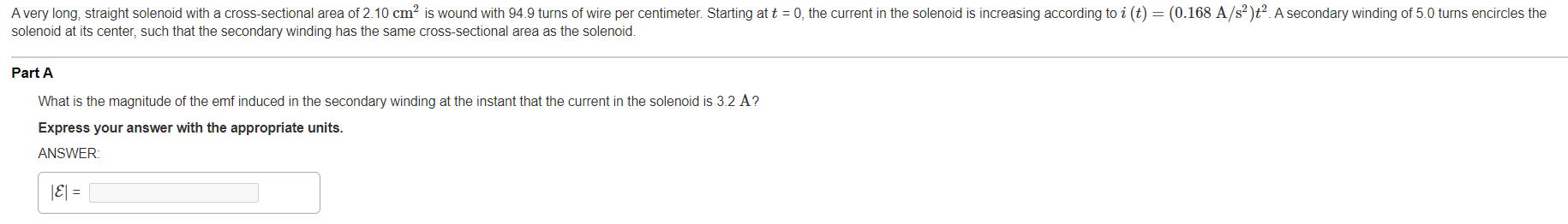A very long, straight solenoid with a cross-sectional area of 2.10 cm² is wound with 94.9 turns of wire per centimeter. Starting at t = 0, the current in the solenoid is increasing according to i(t) = (0.168 A/s²). A secondary winding of 5.0 turns encircles the solenoid at its center, such that the secondary winding has the same cross-sectional area as the solenoid. Part A What is the magnitude of the emf induced in the secondary winding at the...

• ### A very long, straight solenoid with a cross-sectional area of 2.20 cm is wound with 79.0...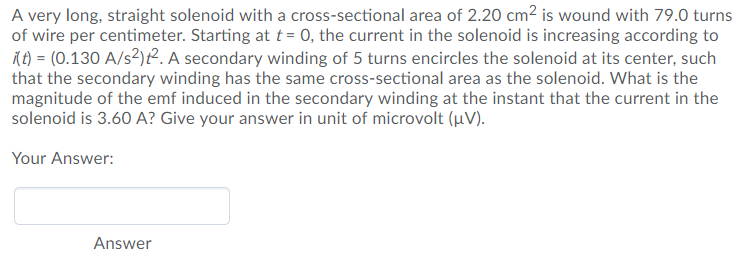A very long, straight solenoid with a cross-sectional area of 2.20 cm is wound with 79.0 turns of wire per centimeter. Starting at t- O, the current in the solenoid is increasing according to t (0.130 A/s2)t2. A secondary winding of 5 turns encircles the solenoid at its center, such that the secondary winding has the same cross-sectional area as the solenoid. What is the magnitude of the emf induced in the secondary winding at the instant that the current...

• ### a solenoid is 20cm long and has a cross sectional area of ​​5x10 ^ -4 m...

a solenoid is 20cm long and has a cross sectional area of ​​5x10 ^ -4 m ^ 2. When the current through the solenoid decreases at a rate of 0.625 A / s, the induced emf is 2x10 ^ -4 V. Find the number of turns / unit length of the solenoid: A. 1825 B. 1325 C. 2050 D. 356 E. 725 F. 1596

• ### A very long, straight solenoid with a cross-sectional area of 6.15 cm² is wound with 48...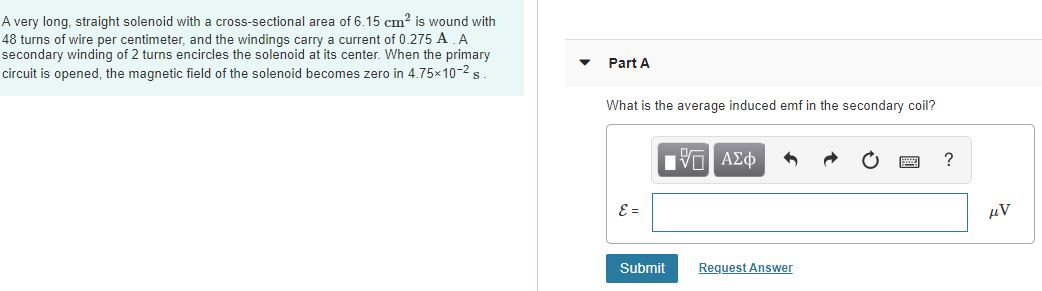A very long, straight solenoid with a cross-sectional area of 6.15 cm² is wound with 48 turns of wire per centimeter, and the windings carry a current of 0.275 A A secondary winding of 2 turns encircles the solenoid at its center. When the primary circuit is opened, the magnetic field of the solenoid becomes zero in 4.75x10-2 s. Part A What is the average induced emf in the secondary coil? IVO ALDA O ? Submit Request Answer

• ### Mutual inductance

A long solenoid (cross-sectional area = 1.0 x 10-6m2 ; 2400 turns/m) is bentinto a circular shap so it looks like a doughnut,called a toroid.Assume that the diameter of the solenoid is small compared tothe radius of the toroid, which is 0.050 m. Find the emf induced inthe toroid whenthe current decreases to 1.1 A from 2.5 A in a timeof 0.15 s.

• ### Problem 29.49 A very long, straight solenoid with a cross-sectional area of 1.80 cm' is wound...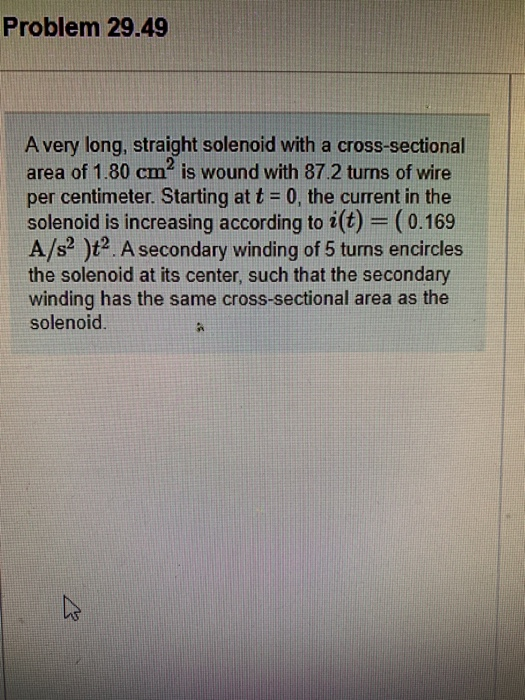Problem 29.49 A very long, straight solenoid with a cross-sectional area of 1.80 cm' is wound with 87.2 turns of wire per centimeter. Starting at t = 0, the current in the solenoid is increasing according to i(t) = (0.169 A/s2 )ta. A secondary winding of 5 turns encircles the solenoid at its center, such that the secondary winding has the same cross-sectional area as the solenoid. Part A What is the magnitude of the emf induced in the secondary...